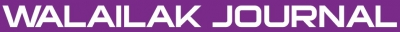A Fractional Finite Difference Method for Solving the Fractional Poisson Equation Based on the Shifted Grünwald Estimate

Abstract

In this study a fractional Poisson equation is scrutinized through finite difference using the shifted Grünwald estimate. A novel method is proposed numerically. The existence and uniqueness of the solution for the fractional Poisson equation is proved. Exact and numerical solutions are constructed and compared. Then numerical results show the efficiency of the proposed method.

Keywords

Fractional Poisson equation, Riemann-Liouville fractional derivative, Shifted Grünwald Estimate, Taylor’s expansion of fractional order

PDF

References

S Momani and Z Odibat. A novel method for nonlinear fractional partial differential equations: Combination of DTM and generalized Taylor’s formula. J. Comput. Appl. Math. 2008; 220, 85-95.

S Momani. A novel Non-perturbative analytical solutions of the space- and time-fractional Burgers equations. Chaos Soliton. Fract. 2006; 28, 930-7.

Q Yang, F Liu and I Turner. Numerical methods for fractional partial differential equations with Riesz space fractional derivatives. Appl. Math. Model. 2010; 34, 200-18.

M Grag and A Sharma. Solution of space-time Fractional Telegraph equation by Adomian decomposition method. J. Inequalities Spec. Funct. 2011; 1, 1-7.

Z Odibat and S Momani. Application of variational iteration method to nonlinear differential equations of fractional order. Int. J. Nonlinear Sci. Numer. Simul. 2006; 7, 15-27.

X Li, M Xu and X Jiang. Homotopy perturbation method to time-fractional diffusion equation with a moving boundary condition. Appl. Math. Comput. 2009; 208, 434-9.

L Song and H Zhang. Application of homotopy analysis method to fractional KDV-Burgers-Kuramoto equation. Phys. Lett. A 2007; 367, 88-94.

A Borhanifar and S Valizadeh. Numerical solution for fractional partial differential equations using Crank-Nicolson method with Shifted Grünwald estimate. Walailak J. Sci. & Tech. 2012; 9, 433-44.

MM Meerschaert and C Tadjeran. Finite difference approximations for two-sided space-fractional partial differential equations. Appl. Numer. Math. 2006; 56, 80-90.

A Borhanifar and MM Kabir. Soliton and periodic solutions for (3+1)-dimensional nonlinear evolution equations by Exp-function method. Appl. Appl. Math. 2009; 229, 158-67.

A Borhanifar, MM Kabir and L Maryam Vahdat. New periodic and soliton wave solutions for the generalized Zakharov system and (2+1)-dimensional Nizhnik-Novikov-Veselov system. Chaos, Solitons & Fractals 2009; 42, 1646-54.

A Borhanifar, MM Kabir. New periodic and soliton solutions by application of Exp-function method for nonlinear evolution equations. J. Comput. Appl. Math. 2009; 229, 158-67.

A Borhanifar, A Zamiri. Application of G'/G-expansion method for the Zhiber-Shabat equation and other related equations. Math. Comput. Model. 2011; 54, 2109-16.

MM Kabir, A Borhanifar and R Abazari. Application of G'/G-expansion method to Regularized (RLW) equation. Comput. Math. Appl. 2011; 61, 2044-7.

A Borhanifar and R Abazari. Application General Solution of generalized (2+1)-Dimensional Kadomtsev Petviashvili (KP) equation by using the G'/G-expansion method. J. Comput. Math. 2011; 1, 219-25.

A Borhanifar and R Abazari. Numerical study of nonlinear Schodinger and coupled Schrodinger equations by differential transformation method. Opt. Commun. 2010; 283, 2026-31.

A Borhanifar and R Abazari. Numerical study of the solution of the Burgers and coupled Burgers equations by a differential transformation method. Comput. Math. Appl. 2010; 59, 2711-22.

P Agrawalar. Solution for a fractional diffusion-wave equation defined in a bounded domain, mechanical engineering and energy processes. Nonlinear Dynam. 2002; 29, 145-55.

J Munkhammar. Riemann-Liouville Fractional Derivatives and the Taylor-Riemann Series. Department of Mathematics Uppsala University. 2004.

I Podlubny. Fractional Differential Equations. Academic Press, New York. 1999.

K Miller and B Ross. An Introduction to the Fractional Calculus and Fractional Differential Equations. Wiley and Sons, New York, 1993.

S Samko, A Kilbas and O Marichev. Fractional Integrals and Derivatives: Theory and Applications. Gordon and Breach, London, 1993.

G Jumarie. Fractional Hamilton-Jacobi equation for the optimal control of non-random fractional dynamics with fractional cost function. J. Appl. Math. Comput. 2007; 23, 215-28.

G Jumarie. Fractional partial differential equations and modified Riemann-Liouville derivative new methods for solution. J. Appl. Math. Comput. 2007; 24, 31-48.

E Isaacson and HB Keller. Analysis of Numerical Methods. John Wiley & Sons Inc., New York, 1966.

Refbacks

• There are currently no refbacks.Online ISSN: 2228-835X

http://wjst.wu.ac.th

Last updated: 17 May 2019